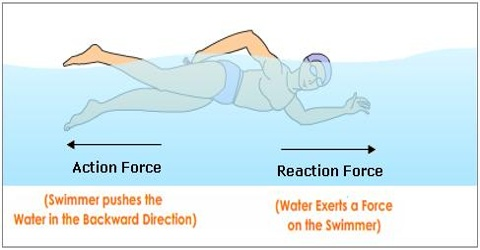# Application of Newton’s Laws of Motion: Towing a Boat

Application/Uses of Newton’s Laws of Motion

When a body applies force on another body then the second body also applies equal and opposite force on the first body. We have learned about this action and reaction forces from Newton’s third law of motion. In nature forces act in couples. In nature, there is not an individual separate force. Two forces are complementary to each other. The normal force on an object is not always equal in magnitude to the weight of the object.

If an object is accelerating, the normal force will be less than or greater than the weight of the object. Also, if the object is on an inclined plane, the normal force will always be less than the full weight of the object. One of these forces is called action force and the other one is the reaction force. As long as the action force is there, there exists a reaction force also. The practical application of Newton’s laws of motion is described below with an example.

Pulling a boat by a rope (Towing a boat):

Once the boat hits the water, we have a new set of physical factors to consider. The most important one is buoyancy. Buoyancy refers to the upward force exerted on an object that is in a fluid. Suppose M is a boat. At point O a rope is fastened and the boat is pulled by the rope along the bank of the river OR with a force F. F can be resolved at point O into two components— horizontal and vertical components [Figure].

Horizontal component = F Cos θ, its direction is along with OA and the vertical component = F Sin θ, its direction is along OB.

An object’s buoyancy depends on the relationship between the object’s weight and the volume of the water it displaces. The upward force exerted on an object is equal to the volume of water it displaces. If the buoyant force is equal to or greater than the weight of the object, the object floats. In physics, this is known as the Archimedes Principle.For a boat to float, enough of the boat’s surface must make contact with the water to generate the proper force of buoyancy. If only a small part of the boat touches the water, the volume of displaced fluid won’t be great enough to counteract the boat’s weight and keep it afloat.

The horizontal component of the force F Cos θ pulls the boat forward and the vertical component F Sin θ pulls the boat towards the bank of the river. But vertical component F sin θ balanced by the helm. Larger the rope of the boat, smaller is the angle θ. consequently, the value of F sin θ will be less and that of F cos θ will be more. So, the boat will move fast ahead.

There are other elements of physics at play when launching a boat. For example, the engine of your vehicle has to generate the right amount of torque to go up the boat ramp. You can also use a combination of physics and trigonometry to figure out the ideal slope for a boat ramp. So when you go boating, remember that you’re really doing science.